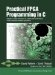# Section 13.3. Creating a Fixed-Point Equivalent

### 13.3. Creating a Fixed-Point Equivalent

The next step is to create a fixed-point equivalent of the same floating-point algorithm. This is important because floating-point math operations are inefficient when rendered in hardware, and also because the C-to-hardware compiler used to create this example does not support floating-point datatypes and corresponding operations.

Converting a floating-point algorithm to a fixed-point equivalent can be a time-consuming process, and the accuracy of the calculations will nearly always result in subtle (and in some cases extreme) differences in behavior. It's critical, then, to perform adequate software simulations to ensure that the fixed-point rendering of the results (including the results of intermediate calculations as appropriate) is of adequate precision for the algorithm in question. The general case of floating- to fixed-point conversion is beyond the scope of this book, but may often involve considerations of rounding (toward infinity and toward 0), saturation, and other factors. The amount of precision required by a given algorithm must also be balanced against the range of numbers to be processed.

For this algorithm we have chosen to use 32-bit fixed-point values, 24 bits of which represent the fractional part. This reflects the fact that the values we will be calculating fall within a narrow range in terms of whole numbers.

The Impulse C libraries include limited support for fixed-point operations, allowing the mandelbrot function to be rewritten as shown in Figure 13-3. (Note that the BMP file header generation section of the fix_mandelbrot function has been removed for brevity.)Practical FPGA Programming in C
ISBN: 0131543180
EAN: 2147483647
Year: 2005
Pages: 208

Similar book on Amazon